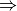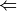# Harmonic Vibrational Analysis, `frequency()` and `hessian()`¶

For further discussion of vibrational and thermochemical analysis, see Sec. Vibrational and Thermochemical Analysis.

`frequency()` is the only command most users will ever need to access directly to perform frequency calculations. Behind the scenes, `frequency()` is a light wrapper over `hessian()` that computes the Hessian then adds a thermochemical analysis.

`frequency`(name[, molecule, return_wfn, func, mode, dertype, irrep])[source]

Function to compute harmonic vibrational frequencies.

Aliases: frequencies(), freq() float – Total electronic energy in Hartrees. (float, Wavefunction) – energy and wavefunction when return_wfn specified. name (string) – `'scf'` || `'mp2'` || `'ci5'` || etc. First argument, usually unlabeled. Indicates the computational method to be applied to the system. molecule (molecule) – `h2o` || etc. The target molecule, if not the last molecule defined. return_wfn (boolean) – `'on'` ||`'off'`Indicate to additionally return the Wavefunction calculation result as the second element (after float energy) of a tuple. Arrays of frequencies and the Hessian can be accessed through the wavefunction. func (function) –`gradient`|| `energy` || `cbs` Indicates the type of calculation to be performed on the molecule. The default dertype accesses `'gradient'` or `'energy'`, while `'cbs'` performs a multistage finite difference calculation. If a nested series of python functions is intended (see Function Intercalls), use keyword `freq_func` instead of `func`. mode (string) –`'continuous'`|| `'sow'` || `'reap'` For a finite difference of energies or gradients frequency, indicates whether the calculations required to complete the frequency are to be run in one file (`'continuous'`) or are to be farmed out in an embarrassingly parallel fashion (`'sow'`/`'reap'`)/ For the latter, run an initial job with `'sow'` and follow instructions in its output file. For maximum flexibility, `return_wfn` is always on in `'reap'` mode. dertype (dertype) –`'hessian'`|| `'gradient'` || `'energy'` Indicates whether analytic (if available- they’re not), finite difference of gradients (if available) or finite difference of energies is to be performed. irrep (int or string) –`-1`|| `1` || `'b2'` || `'App'` || etc. Indicates which symmetry block (Cotton ordering) of vibrational frequencies to be computed. `1`, `'1'`, or `'a1'` represents, requesting only the totally symmetric modes. `-1` indicates a full frequency calculation.

Note

Analytic hessians are not available. Frequencies will proceed through finite differences according to availability of gradients or energies.

```>>> #  Frequency calculation for all modes through highest available derivatives
>>> frequency('ccsd')
```
```>>> #  Frequency calculation for b2 modes through finite difference of gradients
>>> #     printing lowest mode frequency to screen and Hessian to output
>>> E, wfn = frequencies('scf', dertype=1, irrep=4, return_wfn=True)
>>> print wfn.frequencies().get(0, 0)
>>> wfn.hessian().print_out()
```
```>>> #  Frequency calculation at default conditions and Hessian reuse at STP
>>> E, wfn = freq('mp2', return_wfn=True)
>>> set t 273.15
>>> set p 100000
>>> thermo(wfn, wfn.frequencies())
```
`hessian`(name[, molecule, return_wfn, func, dertype, irrep])[source]

Function complementary to `frequency()`. Computes force constants, deciding analytic, finite difference of gradients, or finite difference of energies.

Returns: Matrix – Total non-mass-weighted electronic Hessian in Hartrees/Bohr/Bohr. (Matrix, Wavefunction) – Hessian and wavefunction when return_wfn specified.
```>>> #  Frequency calculation without thermochemical analysis
>>> hessian('mp3')
```
```>>> #  Frequency calc w/o thermo analysis getting the Hessian
>>> #     in file, psi4.Matrix, and np.array forms
>>> set hessian_write on
>>> H, wfn = hessian('ccsd', return_wfn=True)
>>> wfn.hessian().print_out()
>>> np.array(H)
```